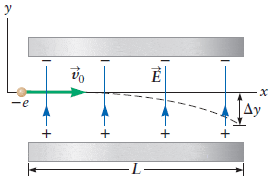# Help: Due Tonight! Electric Field Question

rbraunberger

## Homework Statement

An electron is traveling through a region between two metal plates in which there is a constant electric field of magnitude E directed along the y direction as sketched in the figure below. This region has a total length of L, and the electron has an initial velocity of v0 along the x direction. (Use the following as necessary: e, E, L, m, and v0.)1. By what distance is the electron deflected when it leaves the plates?

2. What is the final velocity (x-direction) of the electron?

F=qE
Motion equations

## The Attempt at a Solution

The questions I have answered.

t= L/v0

F=eE

a=(eE)/m

(F=Electric Force, e=electron charge, E=electric field, m=mass of electron, L=length, t=time)

For the final velocity Vx, I tried to work with the motion equations. Vx=V0+at Vx being final velocity in the x direction, V0 being initial velocity, a is acceleration, and t is time. I plugged in the equations I worked out in the problem for t and a getting Vx=V0 + (eE/m)(L/V0), but the systems says this is an incorrect answer.

For the Distance deflected I also used a motion equation (delta)y=V0 t + 1/2a(y direction) t^2 I worked this down to deltaY= 1/2 (eE/m)(L/v0)^2 and am unsure where to go now.

Thanks for any help!

Last edited:

## Answers and Replies

Homework Helper
welcome to pf!

hi rbraunberger! welcome to pf!i'm not sure what your question is, but the important thing is to find the aceleration …

once you have that, you can treat it much as you would projectile motion under gravityrbraunberger
Thanks, I found the acceleration and was trying to do that....look at my work now...I edited my post since you replied. I have this online homework system and it is not taking my answers....not sure if my logic is just way off.

rbraunberger
Here is a list of all the questions....I am only having trouble with d and first part of e

(a) How long does it take the electron to travel the length of the plates?
t =

(b) What are the magnitude and direction of the electric force on the electron while it is between the plates?
magnitude F =
direction

(c) What is the acceleration of the electron?
magnitude a =
direction

(d) By what distance is the electron deflected when it leaves the plates?
Δy =

(e) What is the final velocity of the electron?
vx =
vy =

Homework Helper
hi rbraunberger!(have a delta: ∆ and try using the X2 and X2 icons just above the Reply box)
For the final velocity Vx, I tried to work with the motion equations. Vx=V0+at Vx being final velocity in the x direction, V0 being initial velocity, a is acceleration, and t is time. I plugged in the equations I worked out in the problem for t and a getting Vx=V0 + (eE/m)(L/V0), but the systems says this is an incorrect answer.

For the Distance deflected I also used a motion equation (delta)y=V0 t + 1/2a(y direction) t^2 I worked this down to deltaY= 1/2 (eE/m)(L/v0)^2 and am unsure where to go now.

hmm … you're throwing v0 and a around like confettiv0 is only in the x direction

a is only in the y direction

start again!rbraunberger
I thought the motion equations were (the ones we are talking about)

Vx = V0 + at
Y=Y0 + V0t + 1/2 at^2

rbraunberger
ok....I removed the a from the X and Vo from the Y and got the answers correct......are the motion equations different in this way with electric fields? My book sucks!!!!

Homework Helper
hi rbraunberger!(just got up :zzz: …)
ok....I removed the a from the X and Vo from the Y and got the answers correct......are the motion equations different in this way with electric fields? My book sucks!!!!

your book does not suck!!this is exactly the same as with projectiles in a gravitational field, isn't it?

you have to remember to deal with the x and y components separately, and that means using only the x or y component of each vector (velocity or acceleration or force) as the case may be …

in the wrong direction, that component is obviously zeroget some sleep! :zzz:​